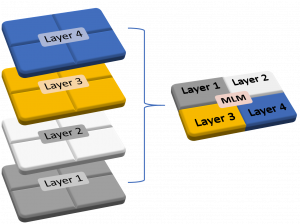# MLM

Together with Multi Project Wafer (MPW), EUROPRACTICE uses the Multi Layer Mask (MLM) technique to reduce fabrication costs.

In this case, the available mask area is typically divided in four quadrants (4L/R : four layer per reticle) whereby each quadrant is filled with one design layer. As an example, one mask can contain four layers such as nwell, poly, ndiff and active. The total number of masks is thus reduced by a factor of four. By adapting the lithographical procedure, it is possible to use one mask four times for the different layers by using the appropriate quadrants. Due to this technique, the mask costs can be reduced by approximately 60%.These are some of the advantages of a MLM run: